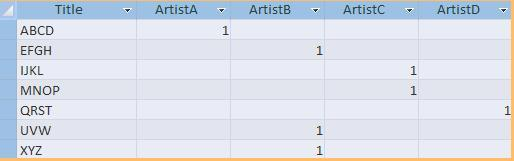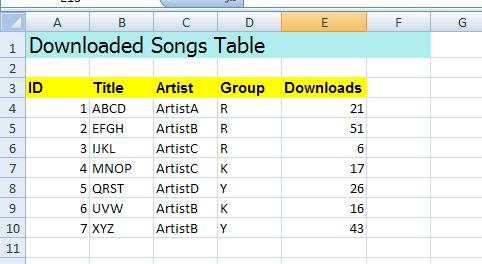Published on
16,118 Points
8,218 Views
4 Endorsements
Approved
The problem in using the built-in Excel generator (TransferSpreadsheet) from Ms Access is that you cannot apply formatting to the cells in Excel during export. The workaround is to create a template first in Excel to use as filename parameter in TransferSpreadsheet. But what if you are generating a dynamic data specially from a crosstab query (variable column).

To solve that, here is a sub procedure I've created in VBA. This is an alternative way to generate dynamic data from MS Access to Excel with some cell formatting.

You can use a Table or non-action Query (such as Crosstab and Select) as your dataSource.

for example you have a crosstab query named as "tablex", with an output as shown below:by calling this subprocedure in VBA or from any control's events (such as command button's click event):

call ExportDataToExcel("Tablex")

it will generate an excel file named as Tablex.xls (or Table.xlsx, depending on what version of Excel you are working on). When you open the excel file, the output should be similar to the figure below:Now here's the code:

In VBA editor (Alt+F11), create a new module then copy the code below.

``````Sub ExportDataToExcel(dataSource As String)
'Requires Reference to Microsoft Excel Object Library
On Error GoTo errExportDataToExcel

Dim rs As Recordset
Dim Xrow, Xcol, rowCtr As Integer
Dim ObjXL As Object
Dim objWkb As Excel.Workbook
Dim objSht As Excel.Worksheet

Set rs = CurrentDb.OpenRecordset("select * from " & dataSource)

If rs.RecordCount > 0 Then

'check & close any instance of Excel running
Set ObjXL = GetObject(, "Excel.Application")
If Not (ObjXL Is Nothing) Then
ObjXL.Workbooks.Close
ObjXL.Quit
Set ObjXL = Nothing
End If

Set ObjXL = CreateObject("Excel.Application")
ObjXL.Visible = False
Set objSht = objWkb.Worksheets(1)
'objSht.Activate

'Put the title first in row 1, column 1
With objSht.Cells(1, 1)
.HorizontalAlignment = xlLeft
With .Font
.Name = "Tahoma"
.FontStyle = "Normal"
.Size = 16
End With
With .Range("A1:F1")
.Merge
.Interior.Color = RGB(175, 238, 238) 'Pale Blue
End With
End With

'Set start of cell to begin data plotting
Xrow = 3
Xcol = 1
rowCtr = 1 'always 1
'plotting of data starts here
Do While Not rs.EOF
'put column name once
If rowCtr = 1 Then
For iField = 0 To rs.Fields.Count - 1
'format the cell first
With objSht.Cells(Xrow, Xcol + iField)
.HorizontalAlignment = xlLeft
.Interior.Color = RGB(255, 255, 0)
With .Font
.Name = "Arial"
.FontStyle = "Bold"
.Size = 11
End With
End With
'then put the column label
objSht.Cells(Xrow, Xcol + iField).Value = rs.Fields(iField).Name
Next
rowCtr = 0
Xrow = Xrow + 1
End If
'actual data plotting
For iField = 0 To rs.Fields.Count - 1
objSht.Cells(Xrow, Xcol + iField).Value = rs.Fields(iField).Value
Next
Xrow = Xrow + 1
rs.MoveNext
Loop

End If

'save the workbook
objWkb.SaveAs CurrentProject.Path & "\" & dataSource
objWkb.Close
'close the workbook
Set objSht = Nothing
Set objWkb = Nothing
ObjXL.Quit
'notify if done processing
MsgBox "Done generating " & dataSource & " to " & CurrentProject.Path
Exit Sub

errExportDataToExcel:
'bypass if error 432 & 429
If Err = 432 Or Err = 429 Then
Resume Next
'otherwise display other error
Else
MsgBox Err & ": " & Err.Description
End If

End Sub
``````

You can use EXCEL's CopyFromRecordset method if you want a faster result, instead of populating one cell at a time. You may replace the codes above, from line 45 to 76 with the codes below:

``````        'Column labels & formatting
Xrow = 3
Xcol = 1
For ifield = 0 To rs.Fields.Count - 1
With objSht.Cells(Xrow, Xcol + ifield)
.HorizontalAlignment = xlLeft
.Interior.Color = RGB(255, 255, 0)
With .Font
.Name = "Arial"
.FontStyle = "Bold"
.Size = 11
End With
End With
objSht.Cells(Xrow, Xcol + ifield).Value = rs.Fields(ifield).Name
Next ifield
'Data population from recordset
objSht.Range("A4").CopyFromRecordset rs
``````

Note:

Before using this function, make sure you have already set reference to the Microsoft Excel Object Library. To set this reference, in VBA Editor, click on the Tools menu, select references, then on the Reference list dialog box, search the item "Microsoft Excel Object Library". Select it by clicking on the check box.

I assume you are already familiar with VBA programming. You may refer to some comments on the above codes to guide you what that block of code does.

With regards to the VBA syntax for Excel's cell formatting, well, I was able to discover those syntax when I tried doing some experiments. Here are some tips:

1. Open an Excel Workbook.
2. Enable the macro record button (upon clicking the record button, the macro will begin recording all your actions on the workbook (like deleting, inserting, merging, coloring cells and even freezing/unfreezing the worksheets).
3. Upon stopping the macro recording (by clicking the stop button), it will automatically create a module that contains the recorded steps that you've made on the workbook, Visual basic langauge.
4. Inspect the codes that were created and only copy what you need.

You may copy it as is and paste it into an Access VB module but it's best to edit the codes based on MS Access coding standards for optimization. Experiment!

Hope this helps.

By the way, if you have questions/clarifications regarding this article, just drop a comment.
4